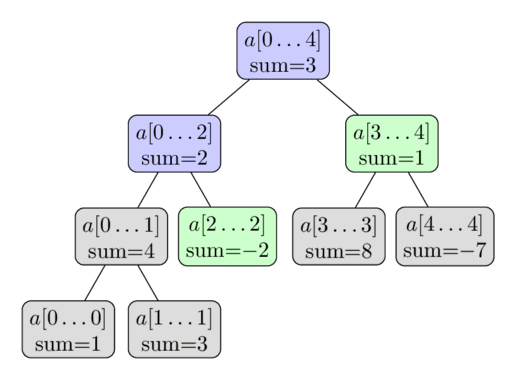# Tutorial : - Easy and Efficient Segment Trees

Don’t worry, it will be easy , I promiseA Segment Tree is a data structure that allows answering range queries over an array effectively, while still being flexible enough to allow modifying the array. This includes finding the sum of consecutive array elements a[l…r], or finding the minimum element in a such a range in O(log⁡n) time. Between answering such queries the Segment Tree allows modifying the array by replacing one element, or even change the elements of a whole sub-segment (e.g. assigning all elements a[l…r] to any value, or adding a value to all element in the sub-segment).

(Source:-Emaxx algos)

Things we can do with a segment tree : -

1)Find sum of elements between 2 indices ‘l’ and ‘r’ of the array .

2)Change any one element of the array, i.e, updating a[i]=x .

We will solve both above queries in O(logn) timeI will discuss about the iterative version which is the easiest to understand :-

Building of segment tree : -

void build(ll a[],ll segtree[],ll n)

{

ll i;

i=0;

while(i<n)

{

segtree[n+i]=a[i];

i++;

}

i=n-1;

while(i>=1)

{ ll pass=2*i;

segtree[i]=segtree[pass]+segtree[pass+1];

i–;

}

i=1;

while(i<2*n)

{

cout<<segtree[i]<<" ";

i++;

}

}

Say, our array is {1,2,3,4,5}

So, our segment tree will look like : - _ , _ , _ , _ , 1 , 2 , 3 , 4 , 5

After sometime, it will look like : -

15,10,5,9,1,2,3,4,5

Easy enough!!

Sum query :-

ll range(ll segtree[],ll left,ll right,ll n)

{

left=left+n;

right=right+n;

ll mi=0;

while(left<right)

{

if(left & 1)

{

mi = (mi + segtree[left]);

left++;

}

if (right & 1)

{

right–;

mi = (mi + segtree[right]);

}

left /= 2;

right /= 2;

}

return mi;

}

Update query :-

You change the actual value, like a[i]=x ; in the segment tree, you do the same, but keep on going above the tree to change the required other values as well(in required ranges)

void update(ll segtree[],ll index,ll value,ll n)

{

index=index+n;

segtree[index]=value; //jahaan__hai___vahaaan___thuso___

while(index>1)

{

index=index/2;

ll kf=2*index;

segtree[index] = segtree[kf] + segtree[kf+1] ;

}

}

Complete implementation : -

Ideone.com

Happy Coding!-kARAN.

3 Likes